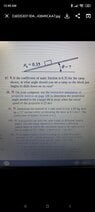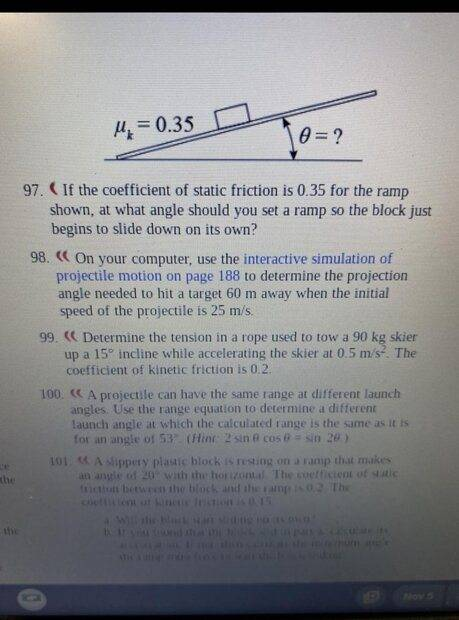# Static and Kinetic Friction -- Tilting a ramp until a block starts sliding

• Alaraity
In summary, the equation for static friction is μ(FS)max = μs N where μs is the coefficient of static friction.

#### Alaraity

Homework Statement
Sf = 0.35

0=?

97. If the coefficient of static friction is 0.35 for the ramp shown, at what angle should you set a ramp so the block just begins to slide down on its own?
Relevant Equations
I break ƩF=ma down to

F(gravity) x sin∅ - μ(kinetic) x F(normal) = ma, trying to find the angle and everything else but I'm stuck, please explain?
None yet

#### Attachments

•Screenshot_2022-11-06-00-45-01-720_com.discord.jpg
20.1 KB · Views: 54
Alaraity said:
Homework Statement:: 14 0.35

0=?

97. If the coefficient of static friction is 0.35 for the ramp shown, at what angle should you set a ramp so the block just begins to slide down on its own?

None yet
"idk" is not adequate. Per forum rules, you must show some attempt. Maybe start by telling us what you understand about the meanings of static and kinetic friction. Any equations related to them?

•Alaraity
I break ƩF=ma down to

F(gravity) x sin∅ - μ(kinetic) x F(normal) = ma, i don't know the angle so I can't find the a

ƩFx = ma = 0
mgsinθ - μmg = 0 are some equationsWelcome @Alaraity !

There is only one force forcing the block to slide on the ramp.
There is only one force resisting that sliding of the block.
Could you identify each force?

Alaraity said:
I break ƩF=ma down to

F(gravity) x sin∅ - μ(kinetic) x F(normal) = ma,
That's true once it is sliding, but the question is asking about the condition to start sliding.
Alaraity said:
mgsinθ - μmg = 0 are some equations
Ok, but it is no use knowing equations if you do not remember when they apply and what all the variables mean. Which friction coefficient is ##\mu## there and in what situation can you use the equation?
Anyway, I don't think that equation is worth learning; it is too particular. You can get it from two more generally useful equations. Do you know an 'equation' (not exactly an equation) regarding the static frictional force?

Edit: Actually it is worse than that. I cannot think of a situation in which that equation is correct.

Last edited:
μ = coefficient of static friction

Alaraity said:
μ = coefficient of static friction
And what about the rest of my questions?

(fs)max = μs N where μs is the coefficient of static friction?

Alaraity said:
(fs)max = μs N where μs is the coefficient of static friction?
It's interesting how many students have a conceptual difficulty with this paticular question and others like it.
Imagine the block is resting on the ramp and you slowly tilt the ramp upwards until eventually the block starts to slide. The question is asking at what angle this happens? In order for the block to slide static friction must be overcome. The kinetic friction will determine the minimum acceleration when the block does start to slide - but it's not relevant to the angle at which the block starts to slide.

Note also that the question is equivalent to asking for the maximum angle at which the block does not slide! That's because we have a threshold angle here. That means there is some angle ##\theta_0## below which the block rests on the ramp and above which the block slides. At the angle ##\theta_0## itself, the forces are still balanced and technically the block does not move.

It might be better, therefore, for the question to ask for the maximum angle at which the block does not slide.

Does that help you to make a better attempt at solving this problem?

•Lnewqban and Lord Jestocost
Alaraity said:
(fs)max = μs N where μs is the coefficient of static friction?
Right, so here's why I did not like your earlier equation, mgsinθ - μmg = 0.
1. The normal force is not necessarily mg. Given that this is on a slope, it might be ##mg\cos(\theta)##, but only if there are no other forces with a vertical component being applied.
2. Equality is only in the case where the system is on the verge of slipping.
So the general statement is
##F_s\leq \mu_sN##
where
- ## N ## is the minimum force necessary to prevent interpenetration of the surfaces in contact. (It follows that it acts perpendicularly to the tangent plane of the contact.)
- A pair of equal and opposite forces ##F_s## acts on the bodies in the tangent plane. On each body it acts in the direction opposing whatever relative motion would otherwise occur.

With that understanding, what equations can you now write for this problem?

•PeroK